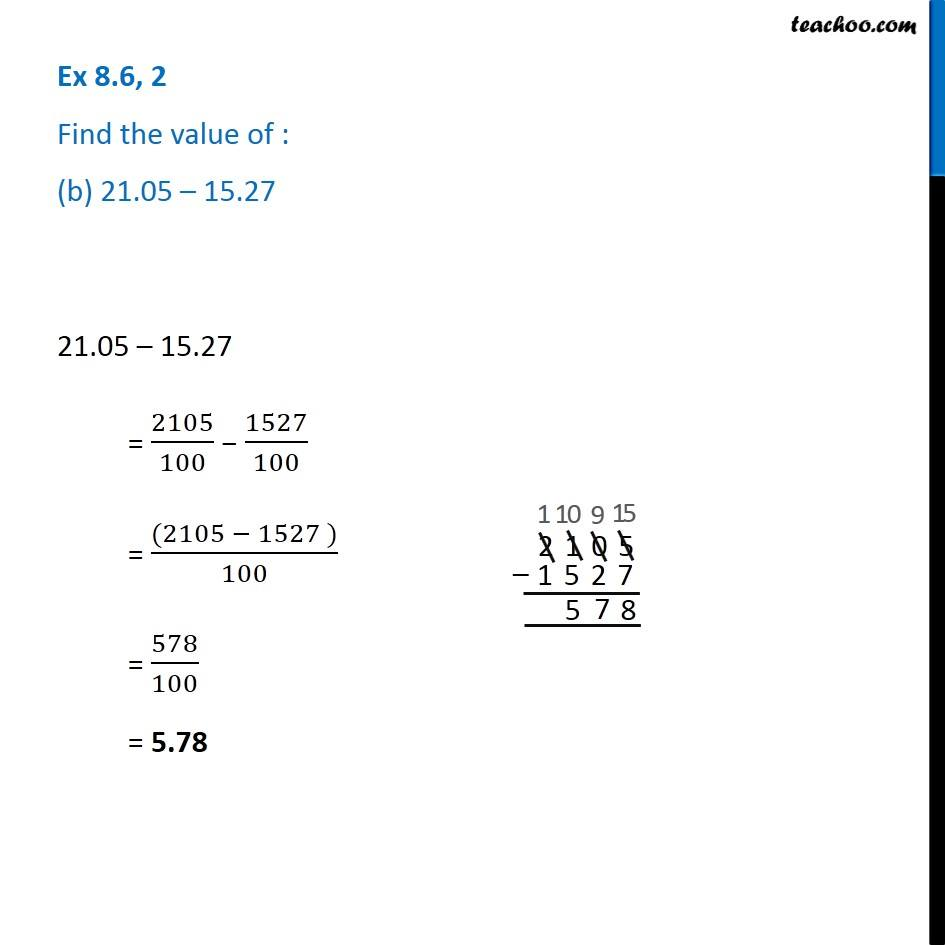1. Chapter 8 Class 6 Decimals
2. Serial order wise
3. Ex 8.6

Transcript

Ex 8.6, 2 Find the value of : (b) 21.05 – 15.27 21.05 – 15.27 = 2105/100 − 1527/100 = ((2105 − 1527 ))/100 = 578/100 = 5.78